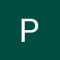Class Central is learner-supported. When you buy through links on our site, we may earn an affiliate commission.

# Mathematical Thinking in Computer Science

### Overview

##### Class Central Tips
Mathematical thinking is crucial in all areas of computer science: algorithms, bioinformatics, computer graphics, data science, machine learning, etc. In this course, we will learn the most important tools used in discrete mathematics: induction, recursion, logic, invariants, examples, optimality. We will use these tools to answer typical programming questions like: How can we be certain a solution exists? Am I sure my program computes the optimal answer? Do each of these objects meet the given requirements?

In the online course, we use a try-this-before-we-explain-everything approach: you will be solving many interactive (and mobile friendly) puzzles that were carefully designed to allow you to invent many of the important ideas and concepts yourself.

Prerequisites:
1. We assume only basic math (e.g., we expect you to know what is a square or how to add fractions), common sense and curiosity.
2. Basic programming knowledge is necessary as some quizzes require programming in Python.

### Syllabus

• Making Convincing Arguments
• Why some arguments are convincing and some others are not? What makes an argument convincing? How can you establish your argument in such a way that there is no room for doubt left? How can mathematical thinking help with this? In this section, we start digging into these questions. Our goal is to learn by examples how to understand proofs, how to discover them on your own, how to explain them, and — last but not least — how to enjoy them: we will see how a small remark or a simple observation can turn a seemingly non-trivial question into an obvious one.
• How to Find an Example?
• How can we be certain that an object with certain requirements exist? One way to show this, is to go through all objects and check whether at least one of them meets the requirements. However, in many cases, the search space is enormous. A computer may help, but some reasoning that narrows the search space is important both for computer search and for "bare hands" work. In this module, we will learn various techniques for showing that an object exists and that an object is optimal among all other objects. As usual, we'll practice solving many interactive puzzles. We'll show also some computer programs that help us to construct an example.
• Recursion and Induction
• We'll discover two powerful methods of defining objects, proving concepts, and implementing programs — recursion and induction. These two methods are heavily used in discrete mathematics and computer science. In particular, you will see them frequently in algorithms — for analysing correctness and running time of algorithms as well as for implementing efficient solutions. For some computational problems (e.g., exploring networks), recursive solutions are the most natural ones. The main idea of recursion and induction is to decompose a given problem into smaller problems of the same type. Being able to see such decompositions is an important skill both in mathematics and in programming. We'll hone this skill by solving various problems together.
• Logic
• Mathematical logic plays a crucial and indispensable role in creating convincing arguments. We use the rules and language of mathematical logic while writing code, while reasoning and making decisions, and while using computer programs. This week we’ll learn the basics of mathematical logic, and we'll practice tricky and seemingly counterintuitive, but yet logical aspects of mathematical logic. This will help us to write readable and precise code, and to formulate our thoughts rigorously and concisely.
• Invariants
• "There are things that never change". Apart from being just a philosophical statement, this phrase turns out to be an important idea in discrete mathematics and computer science. A property that is preserved during a process is called an invariant. Invariants are used heavily in analyzing the behavior of algorithms, programs, and other
processes. Being able to find the right invariant is an important skill that we will develop together in this module.
• Solving a 15-Puzzle
• In this module, we consider a well known 15-puzzle where one needs to restore order among 15 square pieces in a square box. It turns out that the behavior of this puzzle is determined by beautiful mathematics: it is solvable if and only if the corresponding permutation is even. To understand what it means and why it is true, we will learn the basic properties of even and odd permutations — an important notion in algebra and discrete mathematics. Together, we will implement a number of simple methods for working with permutations. You will then use them as building blocks to implement a program that solves any configuration of this game in blink of an eye!

### Taught by

Alexander S. Kulikov, Michael Levin and Vladimir Podolskii

## Reviews

4.5 rating, based on 2 Class Central reviews

4.4 rating at Coursera based on 2058 ratings

Start your review of Mathematical Thinking in Computer Science

•PAZHAMALAI M UEE14227

PAZHAMALAI M UEE14227 completed this course, spending 3 hours a week on it and found the course difficulty to be easy.

Lots of interactive quizzes and challenges makes the course more interesting. More classical problems in computer science, has been explained.
• Anonymous

Anonymous completed this course.

A pretty decent introduction to proofs in general. It covers a basic view of different types of proofs. It's a great introduction to the specialization. Also, it's got a few fun puzzle to solve along with the lectures and quizzes. Pretty neat.

### Never Stop Learning.

Get personalized course recommendations, track subjects and courses with reminders, and more.